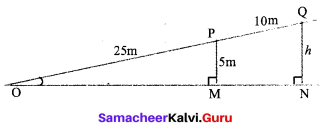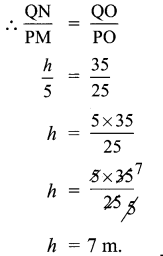You can Download Samacheer Kalvi 9th Maths Book Solutions Guide Pdf, Tamilnadu State Board help you to revise the complete Syllabus and score more marks in your examinations.

## Tamilnadu Samacheer Kalvi 9th Maths Solutions Chapter 6 Trigonometry Ex 6.1

9th Maths Trigonometry Exercise 6.1 Question 1.
From the given figure find all the trigonometric ratios of angle B.
Solution: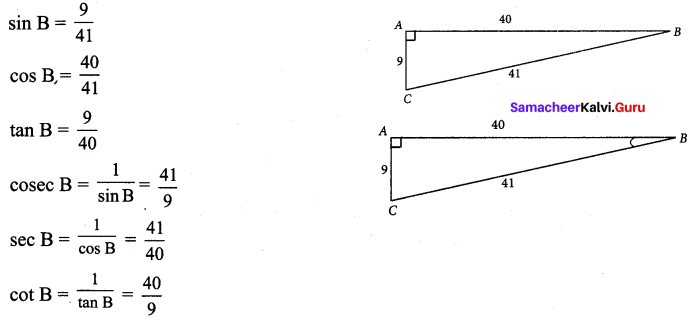9th maths trigonometry exercise 6.1 Question 2.
From the given figure, find the values of
(i) sin B
(ii) sec B
(iii) cot B
(iv) cos C
(v) tan C
(vi) cosec C
Solution: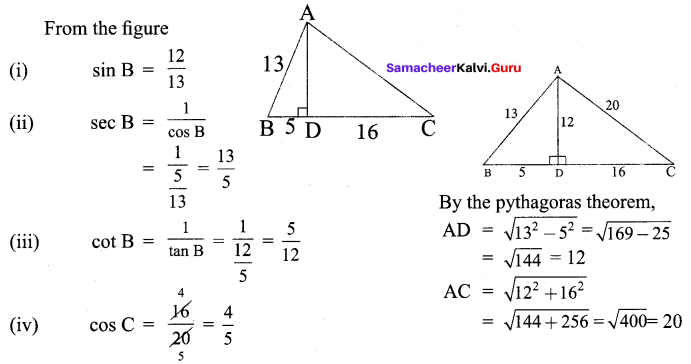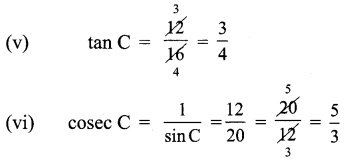trigonometry exercise 6.1 Question 3.
If 2 cos θ = $$\sqrt{3}$$, then find all the trigonometric ratios of angle θ.
Solution: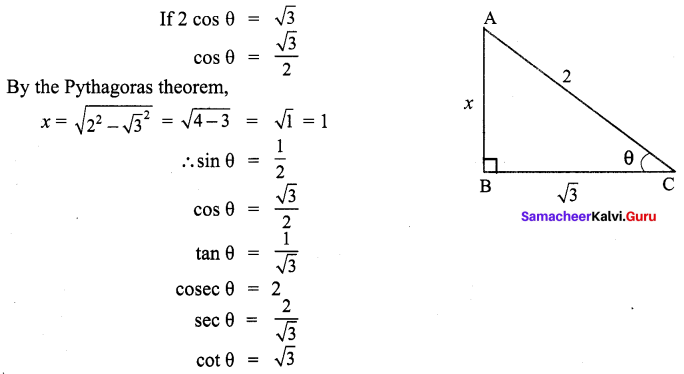Trigonometry solutions exercise 6.1 Question 4.
If cos A = $$\frac{3}{5}$$, then find the value of $$\frac{\sin A-\cos A}{2 \tan A}$$
Solution: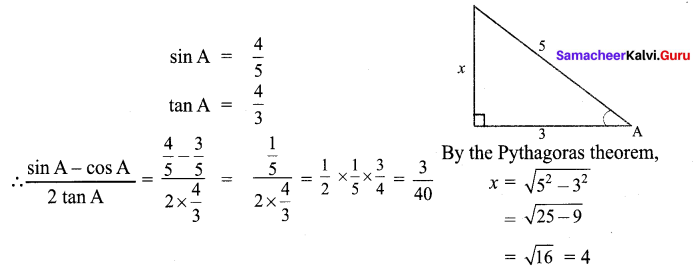trignometry solution of 9th class Question 5.
If cos A = $$\frac{2 x}{1+x^{2}}$$ then find the values of sin A and tan A in terms of x.
Solution: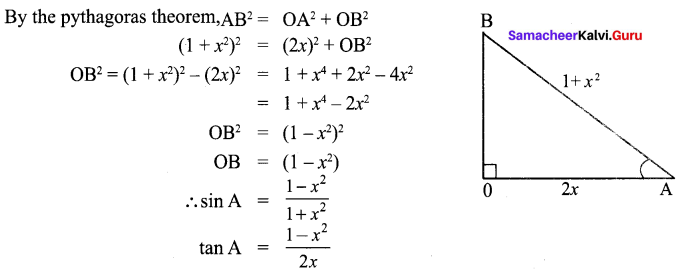9th Maths Exercise 6.1 Samacheer Kalvi Question 6.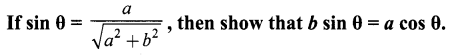Solution: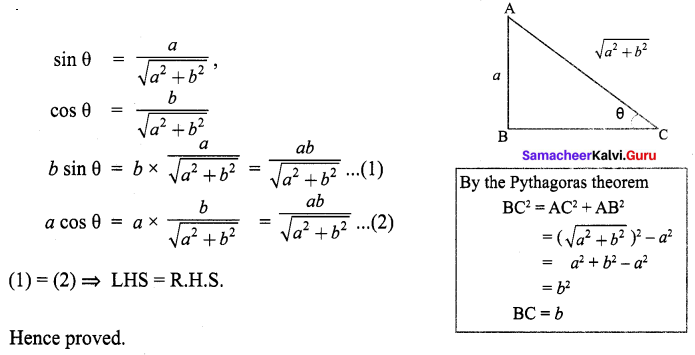9th Maths Exercise 6.1 Question 7.
If 3 cot A = 2, then find the value of $$\frac{4 \sin A-3 \cos A}{2 \sin A+3 \cos A}$$
Solution: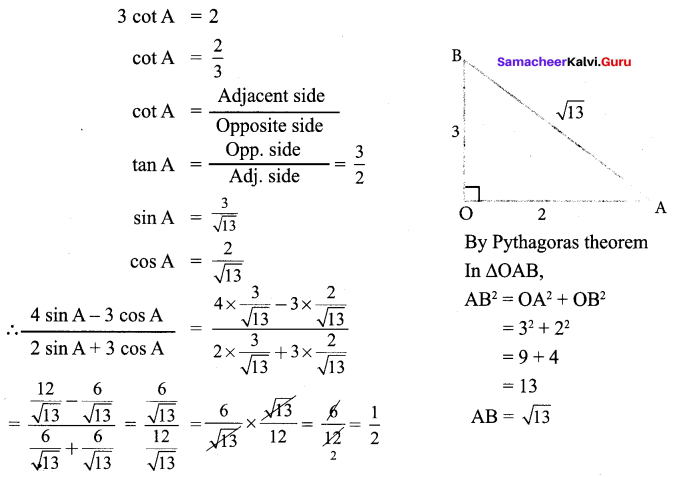9th Std Maths Trigonometry Question 8.
If cos θ : sin θ = 1 : 2, then find the value of $$\frac{8 \cos \theta-2 \sin \theta}{4 \cos \theta+2 \sin \theta}$$
Solution: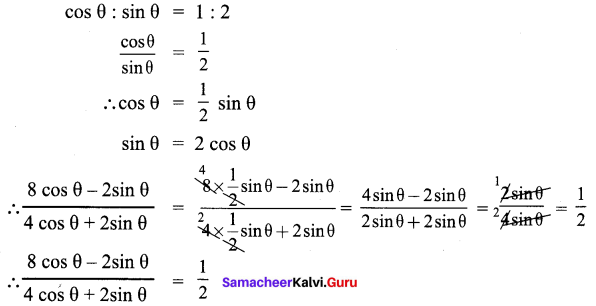9th Maths Exercise 6.1 Solution Question 9.
From the given figure, prove that θ + ϕ = 90°. Also prove that there are two other right angled triangles. Find sin α, cos β and tan ϕ
Solution: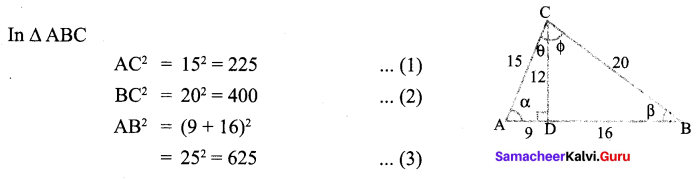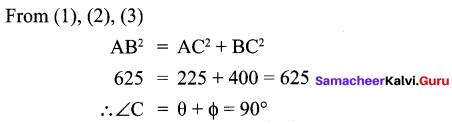(∴ By Pythagoras theorem, in a right angled triangle square of hypotenuse is equal to sum of the squares of other two side)
And also in the figure, ∆ADC, ∆DBC are two other triangles.
As per the data given,
92+ 122 = 81 + 144 = 225 = 152
∴ ∆ ADC is a right angled triangle, then 122 + 162 = 144 + 256 = 400 = 202
∴ ∆ DBC is also a right angled triangle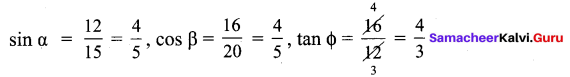9th Maths Trigonometry Question 10.
A boy standing at point O finds his kite flying at a point P with distance OP = 25 m. It is at a height of 5 m from the ground. When the thread is extended by 10 m from P, it reaches a point Q. What will be the height QN of the kite from the ground? (use trigonometric ratios)
Solution:
In the figure,
∆OPM, ∆OQN are similar triangles. In similar triangles the sides are in the same proportional.